### 1. Basis

The two known and one unknown points are the verticies of a plane triangle (not accounting for geodetic effects), Figure C-1.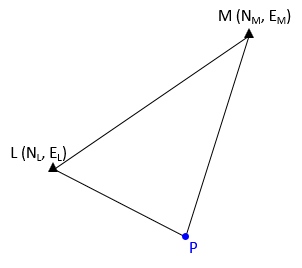Figure C-1 Basic Triangle

Plane trigonometry can be used to solve different triangle parts until enough pieces are known to allow a Forward Computation from one known point or the other.

### 2. Plane triangle formulae

A plane triangle, Figure C-2, has six parts: three angles and three sides.Figure C-2 Plane triangle

Individual angles may be acute (<90°), right (=90°), or obtuse (>90°) with their sum exactly 180°.

To geometrically define a triangle requires three parts, including at least one side, be fixed. Why a side? Because fixing angles alone does not constrain the size of the triangle. The two triangles in Figure C-3 are different sizes even though they have identical angles.Figure C-3 Same angles; different triangle sizes

Equations for triangle trigonometry are:

 Angle condition:Equation C-1 Law of Sines:Equation C-2 Law of Cosines: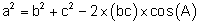Equation C-3

A right triangle is a special case where one angle is exactly 90°. Applying the Law of Cosines, Equation C-3, to a right triangle, Figure C-4:Figure C-4 Right triangleBecause cos(90°) = 0The Law of Cosines becomes the Pythagorean Theorem.

Depending on which parts of it are known the area of a triangle can be determined using one of two equations:

 Using two sides and an included angle:Equation C-4 Using three sides:Equation C-5 where:Equation C-6

### 3. Trigonometric Functions

#### a. Sine; Law of sines

The sine of any angle falls between -1.0 and +1.0. Figure C-5 shows how angles and their sines relate. This curve repeats itself every 360°;  so sin(-340°) = sin(20°) = sin(380°)...Figure C-5

Taking the arcsine (a.k.a. inverse sine, sin-1) of a number between -1.0 and +1.0 on a calculator will always return an angle between -90° and +90°, Figure C-6.Figure C-6 -90° to +90°

Table C-1 shows how angles outside ±90° relate through sin() and sin-1():

 Table C-1 a x = sin (a) b = sin-1(x) relationship 30° +0.500000 +30° b= a 150° +0.500000 +30° a = 180°- b 210° -0.500000 -30° a = 180°- b 330° -0.500000 -30° a = 360° + b

For example sin-1(0.5) = 30° or 150° but it can only be one or the other for a particular triangle. A calculator will always return 30° even though the correct angle may be 150°. How do you know which is correct? It depends on the triangle.

To solve a triangle using the Law of Sines you must have either two angles and a side or two sides and an angle. Moreover, one known side must be opposite a known angle.

##### (1) Example 1

In triangle ABC, a = 12.4', b = 8.7', and B = 36°40'. Compute the remaining angles and side.

From the Law of Sines, Equation C-2:There are two angles whose sine is +0.85112: A = 58°20' and A = (180°00' - 58°20') = 121°40', Figure C-7.Figure C-7 sin-1(+0.85112)

This means there are two possible triangles, Figure C-8.Figure C-8 Two possible triangles

Consider point C as the center of an arc of radius 8.7'.  This arc can intersect the remaining side at two points; A1 and A2, creating two different triangles.

Compute the remaining components for each triangle:

For A = 58°20', Figure C-9:Figure C-9 Law of sines example 1 solution 1

From the angle condition:, Equation C-1:Using Law of Sines:For A = 121°40', Figure C-10:Figure C-10 Law of sines example 1 solution 2

From the angle conditionUsing Law of SinesWhich triangle is the correct solution? It depends on the situation. Notice that the problem statement provided numbers but not a sketch. Had a sketch been included, then the correct triangle could have been selected.

##### (2) Example 2

Compute angle B in the triangle of Figure C-11.Figure C-11 Law of sines example 2

The missing angle can be computed two ways:

- By Law of Sines- By angle conditionAs a result there are two “correct” answers for the missing angle;

sin(108°) = sin(72°) = 0.95105652

But only 108° fits both the Law of Sines and the Angle condition.

We can also visually determine which angle is correct, providing we have a reasonably drawn sketch. In Figure C-11 angle B is larger than 90° so we would select 108°.

 Be careful when using the Law of Sines to solve for an unknown angle – there could be two possible answers only one of which will fit the particular triangle.

#### b. Cosine; Law of cosines

The cosine of any angle falls between -1.0 and +1.0. Figure C-12 shows how angles and their cosines relate. This curve repeats itself every 360°;  so cos(-340°) = cos(20°) = cos(380°)...  The cosine curve is identical to the sine curve except its phase differs by 90°.Figure C-12

Taking the arccos (a.k.a. inverse cosine, cos-1) of a number between -1.0 and +1.0 on a calculator will always return an angle between 0° and 180°, Figure C-13.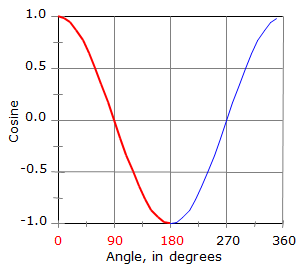Figure C-13 0° to 180°

Table C-2 shows how angles outside ±180° relate through cos() and cos-1():

 Table C-2 a x = cos(a) b = cos-1(x) 60° +0.500000 +60° 120° -0.500000 +120° 300° +0.500000 +60° -240° -0.500000 +120°

Using the Law of Cosines will not cause an ambiguous solution as does the Law of Sines since any single angle in a triangle cannot exceed 180°.

To solve a triangle using the Law of Cosines you must have either three sides, or, two sides and an angle.

##### (1) Example

Compute the value of the angle R in the triangle of Figure C-14.Figure C-14 Law of cosines example

From the Law of Cosines:The Law of Cosines returns only one legitimate value when solving triangles.

#### c. Tangent

Unlike sine and cosine, the tangent of any angle not limited to the range of -1.0 to +1.0. As a matter of fact, the tangent range is ±(infinity). You can see that the tangent function plot, Figure C-15, is not sinusoidal as are the sine and cosine plots. And unlike the other two it repeats itself every 180°;Figure C-15

The tangent curve is asymptotic at 90°, 270°, 450°, etc. Asymptotic means the curve gets close to, but never reaches, those values. Recall that tangent is sine divided by cosine. At 90°, sine = 1.0, cosine = 0.0, so tangent = 1.0/0.0 (with an identical pattern every 180°). The result of dividing by zero is infinity, hence the asymptotic plot.

Try evaluating tan(90°) on your calculator; you’ll probably get an error statement of sorts. Then try tan(89.99999°); you should get a pretty big number.

There is a Law of Tangents, but we don't generally use it to solve triangles since the Laws of Sines or Cosines are usually sufficient .

### 4. Solving triangles

If we start with three triangle parts (including at least one distance), we can solve the reaming three parts using combinations of the Law of Sines and Law of Cosines.
Usually (although not always) there is only one way to start solving a triangle. With each additional part, there are more ways to compute remaining pieces.

Figures C-16 and C-17 show different initial angle and distance combinations and the logic to solve remaining trianle parts.Figure C-16 Example triangle 1 Given: Sides a, b, and c Compute: (1) angle A using Law of Cosines. (2) angle B using Law of Cosines or Law of Sines. (3) angle C using Law of Cosines or Law of Sines or angle condition.Figure C-17 Example triangle 2 Given: Sides b, c and included angle A. Compute: (1) side ausing Law of Cosines (2) angle Busing Law of Cosines or Law of Sines. (3) angle Cusing Law of Cosines or Law of Sines orangle condition

Try figuring out the logic to solve triangles in Figures C-18 through C-21 on your own.Figure C-18 Example triangle 3 Given: Angles A, C and side b.Figure C-19 Example triangle 4 Given: Angles A, C, and side a.Figure C-20 Example triangle 5 Given: Sides c, b, and angle CFigure C-21 Example triangle 6 Given: Angles A, B, and C

### 5. Intersections

#### a. Solution logic

Determining the position of an unknown point requires a combination of triangle solutions along with inverse and Forward Computations. Two points with known coordinates (A and B) form one side of a triangle; inversing between them gives the length and direction of that side (the base line), Figure C-22.Fig C-22 COGO triangle

Recall that three parts of a triangle, including one side, must be known to compute the other parts. Performing an Inverse Computation between the two known points fixes one side of the triangle. The other two parts come from the measurements connecting the unknown point with the base line. Using the Law of Sines or Cosines, enough additional triangle parts are computed to connect the unknown point to a known point with a distance and direction. The unknown coordinates can then be computed using a Forward Computation.

In each of the following intersection descriptions:

• Points J and K have known coordinates and define the base line
• P is the unknown point whose position is being determined

The first step in each intersection is to inverse between points J and K to determine the base line's length and direction, thus fixing one side of the triangle and its orIentation with respect to north. The final step is a Forward Computation from one end of the base line to the unknown point. Either end of the base line may be used, computing from the other could serve as a math check.

#### b. Distance-distance

Distances from points J and K to point P (dJ and dK) are known, Figure C-23.Figure C-23 Distance-distance intersection

A distance-distance intersection results in two possible locations, Figure C-24.Figure C-24 Two intersection points

Triangles JKP1 and JKP2 are mirror images: they have identical angles and distances. It's up to the surveyor to decide which point intersection point, P1  or P2 , to be solve. This mirror triangle situation is the reason it's difficult to determine the unknown position using simultaneous solution of Equations B-1 and B-2. The azimuth from Point J to point P is:Equation C-7

where J is the angle at point J between the base line and the line to point P.

It's relatively simple using triangle equations to solve for point P once we know which side of the base line it is.

We'll solve for point P1, Figure C-25, using a Forward Computation from point J at the base line end. We already know the distance dJP, the direction AzJP is needed.Figure C-25 Distance-distance solution

Solution process

Set up the Law of Cosines and rearrange it to compute angle Q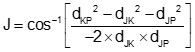Compute azimuth, AzJP , from point J to the intersection point. Because point P is to the left of the base line, Equation C-7 becomes:Forward compute from point JWhat would change had we wanted the position of point P2 instead of point P1? Angle J would be the same except it's right of the base line instead of right. Equation C-7 would be:In order for there to be an intersection point, (dJP + dKP) must be greater than dJK. If (dJP + dKP) = dJK then the intersection point is on the base line and the trianlge is a straight line, Figure C-26.Figure C-26 Distance-distance single intersection condition

#### c. Direction-distance

Direction from one control point (AzJP), and distance from the other (dKP) are known, Figure C-27.Figure C-27 Direction-distance intersection

Let's determine the position of point P using a Forward Computation from point J. To do so, we need the distance dJP. We know two sides (dJK and dKP) and the angle at point J which can be calculated from the two directions AzJK and AzJP. The simplest way to sovle dJP is using the Law of Sines which requires the angle at K.

The solution process is:

Compute angle J by finding the difference between the base line direction (AzJK) and direction to Point P (AzJP). The smaller direction is subtracted from the larger. In this case:Determine the angle at P using the Law of SinesRemember that we have to be careful using the Law of Sines to compute an angle because it is ambiguous: the angle can be P or 180°-P. Figure C-28 shows the two different possible triangles. Distance dKP can intersect the direction AzJP at two locations.Figure C-28 Direction-distance two intersections

We have to pick point  P1 or  P2 based on whether the angle P is acute (<90°) or obtuse (90°) which depends on the particular situation.

Once the correct angle P is selected, angle K is computed from the angle conditionDistance  dJP is determined from the Law of SinesFinally, forward compute from point J to get the coordinates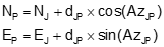If  angle P is exactly 90° a direction-distance intersection will have only a single intersection point, Figure C-29.Figure C-29 Direction-distance single intersection condition

#### d. Direction-direction

Directions from points Q and R to point P (AzQ, and AzR) are known, Figure C-30.Figure C-30 Direction-direction intersection

Two directions intersect at only a single point so there are no multiple solutions for this method. We'll look at computing point P's coordinates as a Forward Computation from point J.

The process is:

Compute angle J as the difference between AzJP and base line azimuth AzJK

Compute angle K as the difference between AzKP and base line back-azimuth AzKJ = (AzJK±180°)

Compute angle P using the angle condition.Use Law of Sines to solve distance from point J to point PForward compute from JThe closer AzJP and AzKP values are to each other, the further away their intersection will be; if the two azimuths are parallel, then no intersection is possible, Figure C-31.Figure C-31 Parallel directions: no intersection

Direction-direction is the only method which has a single intersection point. The other two methods involve at least one distance which result in two possible intersections.